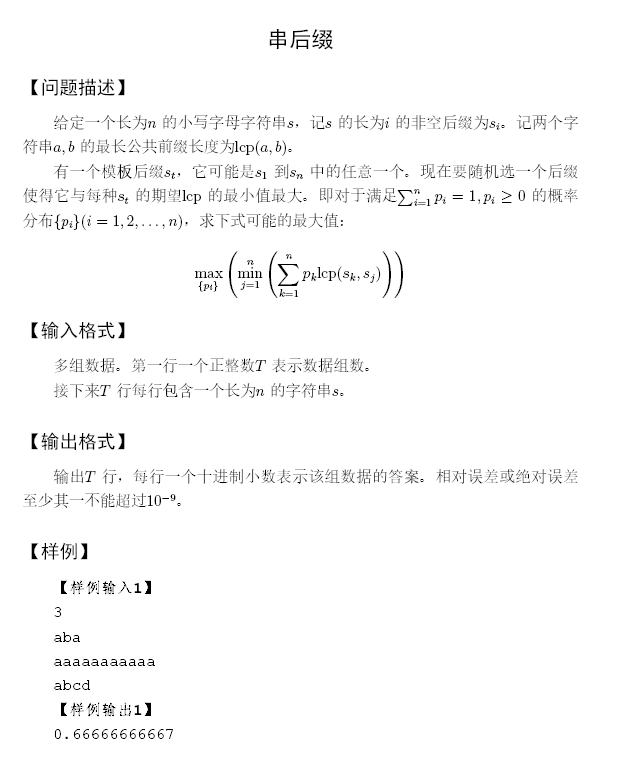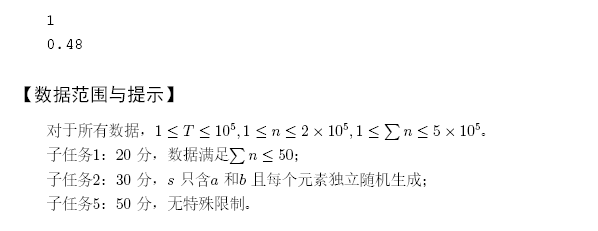# 题意：# 题解：

（dllca膜你赛搬原题差评）

$$\begin{cases}\sum\limits_{i=1}^{n}p_i=1 \\ p_1l_1=p_2l_2=\cdots=p_nl_n=k\end{cases}$$

$$k=\frac{1}{\frac{1}{l_1}+\frac{1}{l_2}+\cdots+\frac{1}{l_n}}$$

dfs的时候注意如果一个节点本身已经是原串某一个后缀的尾节点那么它的后继节点肯定没有贡献，直接返回0即可；

# 代码：

 1 #include<algorithm>
2 #include<iostream>
3 #include<cstring>
4 #include<cstdio>
5 #include<cmath>
6 #include<queue>
7 #define inf 2147483647
8 #define eps 1e-9
9 using namespace std;
10 typedef long long ll;
11 typedef double db;
12 struct edge{
13     int v,next;
14 }a;
16 char s;
18     a[++tote].v=v;
21 }
22 void extend(int ch){
23     int p=last,np=++tot;
24     mx[np]=mx[p]+1;
25     for(;p&&!son[p][ch];p=fa[p])son[p][ch]=np;
26     if(!p)fa[np]=rt;
27     else{
28         int q=son[p][ch];
29         if(mx[q]==mx[p]+1)fa[np]=q;
30         else{
31             int nq=++tot;
32             mx[nq]=mx[p]+1;
33             memcpy(son[nq],son[q],sizeof(son[q]));
34             fa[nq]=fa[q];
35             fa[q]=fa[np]=nq;
36             for(;p&&son[p][ch]==q;p=fa[p])son[p][ch]=nq;
37         }
38     }
39     isp[np]=true;
40     last=np;
41 }
42 db dfs(int u){
43     if(isp[u])return 0;
44     db ret=0;
46         int v=a[tmp].v;
47         ret+=1.0/(dfs(v)+mx[v]-mx[u]);
48     }
49     return 1.0/ret;
50 }
51 int main(){
53     scanf("%d",&t);
54     while(t--){
55         scanf("%s",s);
56         n=strlen(s);
57         rt=last=++tot;
58         for(int i=n-1;i>=0;i--){
59             extend(s[i]-'a');
60         }
61         for(int i=rt+1;i<=tot;i++){
67 }